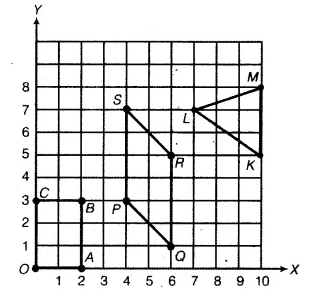# Write the coordinates of the vertices of each of these adjoining figures

Write the coordinates of the vertices of each of these adjoining figures.Clearly, from the graph the coordinates of points are :
O(0, 0), A(2, 0), B(2, 3) and C(0, 3);
P(4, 3), Q(6, 1), R(6, 5) and S(4, 7);
L(7, 7), M(10, 8) and k(10, 5).